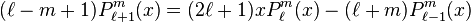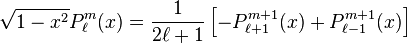## Sunday, September 6, 2015

### ## Spherical Harmonics

The climate blogging scene seems to be running out of steam. WUWT is mainly the same old posters with the same stories. Climate Audit blogs about football aeration. Lucia blogs about just about anything. So it seems an alternate topic is needed. I'll keep blogging about climate science, of course, but also maybe a bit about maths. It won't be click bait, but I hope there will be things worth discussing.

The maths for today is at least something I've been using, and I'll post a file which may be useful. I'm talking about spherical harmonics. These are functions orthogonal on a sphere, which can be used in the same way as you might use Fourier series. I use them for smoothing, and I'll be using them in future for integration.

I'll talk a little bit about where they come from - you can skip if you like. Then I'll show a method I now use for calculating them, with an associated table of coefficients that you can download.

The reason I've been looking at the methods aspect is that I wanted to do a Javascript implementation, for interactive use. I have long had a R implementation, which I've used for TempLS output. I use recurrence relations, to be described. It's not particularly hard to program, but easy to make mistakes. So, as I often do nowadays, I thought to pack the logic into a table of numbers which could be used with a simple mechanical program in any platform. I'll link the table. In the section below, you can skip all the math, which is not compulsory, and go straight to usage.

#### Origins

Spherical harmonics are eigenfunctions of the Laplacian on the sphere. That is, they are solutions of
2Ψ = λΨ for some λ, on the whole sphere (no boundaries).

The reason they are orthogonal is similar to the orthogonality of eigenvectors of symmetric matrices (the Laplacian is self-adjoint). If
2Ψ1 = λ1Ψ1 and ∇2Ψ2 = λ2Ψ2, then integrating over the sphere
λ1∫ Ψ1 Ψ2 = ∫ ∇2Ψ1 Ψ2
= -∫ ∇Ψ1 . ∇ Ψ2 integrating by parts
= ∫ Ψ12Ψ2
= λ2∫ Ψ1 Ψ2

So if λ1 != λ2, then
∫ Ψ1 Ψ2 = 0

#### Separation of variables

Using a coordinating system of the sphere consisting of latitude θ and longitude φ, we can use separation of variables to get two separate ordinary differential equations, for which θ and φ are eigenfunctions. The equation for φ is just the simple harmonic with trig function solutions. The equation for θ is a Legendre equation, for which the solutions are Associated Legendre polynomials Plm(sin(θ)). Together, the spherical harmonics are

Ylm=N Plm(sin(θ)) exp(i m φ) for l=0,1,2..., m=-l,...l, N a normalizing factorVisual representations of the first few real spherical harmonics. Blue portions represent regions where the function is positive, and yellow portions represent where it is negative. The distance of the surface from the origin indicates the value of in angular direction (Wiki)

You can think of it as a table, with Y00=1 at the top, m as the x variable and l as -y. This is the table that I compute.

A comment on the symmetries. As l increases, down the centre, each row brings an extra layer on the axis. As you go from that axis, you get one extra period around the equator, and one less along the axis. So the fourth row (l=3) end has three periods around the equator, and none along the polar axis. This increase of oscillations translates into ability to resolve variation. If you go to row 13, the period is about 30 degrees, so you can resolve variation on that scale.

#### Recurrence relations and array

Being essentially hypergeometric, there is a rich collection of three-term recurrence relations. I number the table by increasing m and then by L, ie by rows. I can compute any term provided I have a recurrence relation connecting it to two already known values. As an integer array, I can take any value out of the displayed cone (l≥0, |m|≤l) to be zero.

For most, I use the relation connecting three consecutive l values (x=sin(θ)):But this fails when m=l+1 - ie at end of rows. So then I userelying on the last term being zero.

There are some variations. I don't use complex values, but rather the trig component cos(mφ) for m>0, and sin(mφ) for m<0. I calculate the trig formulae by summation, so that makes the edge formulae three-term again. And I modify the reduced relation to
Pm+1m+1 = -cos(θ) Pmm
absorbing the (2m+1) factor into the normalizer N.

#### Usage

So after all that, I assemble a table that I call hm, with 9 rows, and as many columns as harmonics required. If you include n rows from the table, there are n2 terms, and that number of columns required. The 9 rows are:
• l
• m
• i2 pointer to recurrence location 3
• i1 loc 2
• j whether to use sin(θ) etc in recurrence
• c2 third coef in reccurence
• c1 second
• c0 first
• N normalizer.

So the implementation is just, successively, column i by column, H(1)=1,
c0 H(i)= s(j) c1 H(i1) + s(j+1) c2 H(i2)
s is an array consisting of (sin(θ), 1, cos(θ)cos(φ), cos(θ)sin(φ)), so j will be 1 or 3 (for row ends).

Here is a csv file of that table (transposed), with 441 columns, equivalent to 21 rows.

Here is an R usage:

```hm=read.csv("https://s3-us-west-1.amazonaws.com/www.moyhu.org/blog/math/SHcoef.csv")

makeSH=function(la,lo,N){#Forms spherical harmonics YLM by implementing HM.
# la, lo are lat/lon in degrees
n2=(0:N+1)^2
N1=max(n2)
H=matrix(1,length(la),N1) # will be SH array
p2=pi/180
ss=cbind(sin(la*p2),1,cos(la*p2)*sin(lo*p2),cos(la*p2)*cos(lo*p2));   #
for(i in 2:N1){
m=hm[,i]; # hm is the table of coefs
H[,i]=(H[,m]*m*ss[,m]+H[,m]*m*ss[,m+1])/m
}
for(i in 1:N1)H[,i]=H[,i]*hm[9,i]
H
}
```

You supply a set of lat/lon in degrees, and N+1 for number of rows, and you get an array H of orthonormal harmonics.

#### More usage

So what to do with it? Given any set of points (stations) on the sphere, and values v, you can project, regression wise:

b = (HTH)-1(HTv)

for the coefficients, and then

V = H b

is the SH approximation. You can pick out terms or just display the smoothed approx. I am more enthusiastic now about using this for integration, when the coefficient b1 is the approximate integral (since H1 normalized is 1/4π and all other terms have zero integral. I think this method is almost as good as the mesh methods I use, and a lot faster (if stored weights are unavailable).

1.We use spherical harmonics in X-ray crystallography too, but for a different purpose - orientation searching.

Sometimes you have to similar proteins which crystallize in different orientations. The diffraction pattern will then show the same features, but in a different orientation. (We also essentially see the diffraction pattern sampled on a lattice, which is the Fourier transform of the crystal lattice). We want to match up the diffraction patterns in order to use one known protein to fill in missing information for the unknown protein.

You can do a translation search efficiently using Fourier transforms to calculate the convolution of one 3D field with another (or rather its inverse), giving you the translation which optimally matches on field onto the other. It turns out that you can do the same thing with spherical harmonics to determine the optimum rotation to match one field onto another. It exploits the same property - that spherical harmonics are orthogonal, in the same way that Fourier terms are.

Kevin C

2.At one time, you were considering modifying TempLS to generate temperature reconstructions. Still in the cards?

1.cce,
I looked at it. But the main thing is that the issues with paleo are not spatial accuracy. That's not to say that spatial information is good; on the contrary, it means that there may not be enough to gain from better interpolation.

Improved integration works when there is enough spatial correlation to interpolatre between sites. With paleo, we generally don't know much about the correlation.

The other issue was that TempLS is oriented toward month/year data. Everything is simpler in the new version, so that is just a small barrier to be overcome.

3.Sorry, didn't mean this as it relates to interpolation. I was thinking more along the lines of, "if you need something to blog about, there's that." You did some great work with the Marcott emulation. Replicating (or not) some of the other reconstructions could be a cottage industry with the right tools.

4.That's a very clean implementation of spherical harmonics.

I hadn't seen that particular Wikipedia illustration, which very nicely exhibits the symmetries involved.

1.Thanks, Carrick. I'm hoping to post a local illustration soon.

5.The earth's spherical symmetry is an excuse for many scientists to punt at the idea of working out simplified standing wave models for climate indices such as ENSO.
6.1.